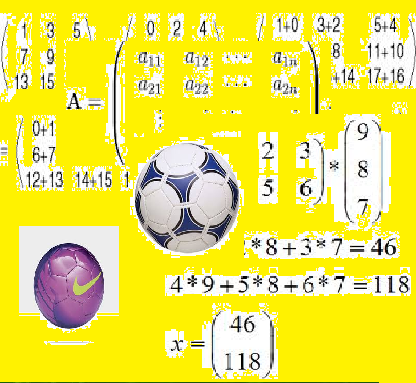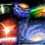# Approaching to Matrices in a different way.Friends this is a problem i had posted two weeks ago maybe . Did anyone find a pragmatic solution. This question is from the AITS which fiitjee conducts unfortunately i don't have the hints and solutions.I only know the answer.Do participate in this discussion. and try to help me out.

A matrix $H=[h_{jk}]$ is used to keep track of three football players (numbered 1,2 and 3) in three matches (1st,2nd and 3rd).

$Re(h_{jk})=A$ A= number of matches in which both jth and kth players played or both did not play.

If j=k,A=3

$Img(h_{jk})=B$

B = (number of matcher played by kth player) - (number of matches played by jth player)

Let$P=[P_{jk}]$ $P_{jk}=C$ C=1 if jth player played in kth match and C=i otherwise $i=(-1)^{0.5}$

$det(H)=D$ $\left| D\right|=E$

Only in the answer two parallel lines represent det and not absolute value.

The answer is D is a non negative multiple of four. but how???????Note by Milun Moghe
7 years, 5 months ago

This discussion board is a place to discuss our Daily Challenges and the math and science related to those challenges. Explanations are more than just a solution — they should explain the steps and thinking strategies that you used to obtain the solution. Comments should further the discussion of math and science.

When posting on Brilliant:

• Use the emojis to react to an explanation, whether you're congratulating a job well done , or just really confused .
• Ask specific questions about the challenge or the steps in somebody's explanation. Well-posed questions can add a lot to the discussion, but posting "I don't understand!" doesn't help anyone.
• Try to contribute something new to the discussion, whether it is an extension, generalization or other idea related to the challenge.

MarkdownAppears as
*italics* or _italics_ italics
**bold** or __bold__ bold
- bulleted- list
• bulleted
• list
1. numbered2. list
1. numbered
2. list
Note: you must add a full line of space before and after lists for them to show up correctly
paragraph 1paragraph 2

paragraph 1

paragraph 2

[example link](https://brilliant.org)example link
> This is a quote
This is a quote
    # I indented these lines
# 4 spaces, and now they show
# up as a code block.

print "hello world"
# I indented these lines
# 4 spaces, and now they show
# up as a code block.

print "hello world"
MathAppears as
Remember to wrap math in $$ ... $$ or $ ... $ to ensure proper formatting.
2 \times 3 $2 \times 3$
2^{34} $2^{34}$
a_{i-1} $a_{i-1}$
\frac{2}{3} $\frac{2}{3}$
\sqrt{2} $\sqrt{2}$
\sum_{i=1}^3 $\sum_{i=1}^3$
\sin \theta $\sin \theta$
\boxed{123} $\boxed{123}$

Sort by:

I think every one should discuss and maybe we can find out a solution.

- 7 years, 5 months ago

From the answer I came to a conclusion of proving that H=PP'. Where P'. is the transpose conjugate of P but how can we prove this?

- 7 years, 5 months ago